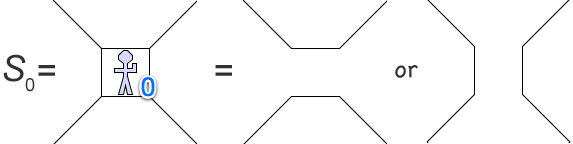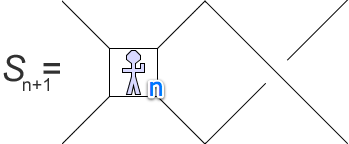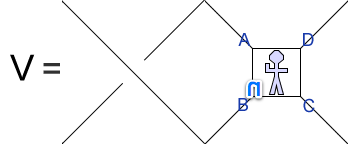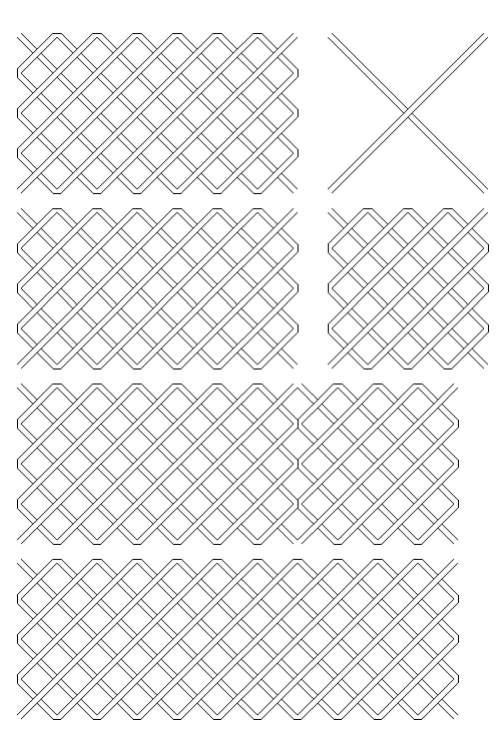# Symmetric Tangles

##### Age 14 to 16

Published 2007 Revised 2019

In Twisting and Turning we introduced you to the Conway Rope Trick. You'll need to take a look at the video on that page and do the rope trick for yourself before reading the rest of this article, since here we're going to take a good long look at the symmetries of the resulting tangles. It may seem very surprising that tangles do have symmetries, so to start off I suspect we need to see an example.

Please note that this video has no sound.

If you can't access YouTube, here is a direct link to the video: Tangle Symmetry.mp4

It does appear to be possible to manipulate the tangle in the video into a form where it has rotational symmetry of order 2 about all three axes.

Could this be true for any tangle made by the Conway Rope Trick?

You may also have noticed that two consecutive turns have no effect on the tangle number. For example, the tangle 4/3 becomes -3/4 after one turn, but a second turn takes it back to 4/3. This only makes sense if all tangles really do have rotational symmetry of order 2 about their turn axis (the axis perpendicular to the plane of the ropes).

What about the rotational symmetry about the other axes? Is this implied by the tangle operations? Well, if we start at 4/3 again, twist once to 7/3, turn once to -3/7, again to 7/3, and then untwist we're back at 4/3. The rope ends will, however, have undergone a rotation of 180 degrees about an axis parallel to the rope plane. You should be able to find a similar sequence that shows that the third axis must also be an axis of symmetry.

It seems that the Conway Rope Trick can only work if it is indeed always true that the tangles it generates - the rational tangles - have rotational symmetry of order 2 about 3 perpendicular axes. Let's see if we can sketch out a proof that these symmetries must all be present using induction !

To make induction work, we're going to have to do three things:

1. Label rational tangles with an integer n so that as n increases from zero, we can be sure that all the rational tangles are included.
2. Show that tangles labeled with n = 0 have the required symmetries.
3. Show that if all tangles labeled with n have the required symmetries, then so do all tangles labeled with n +1.

#### Step 1: Labeling

One way to label all tangles with an integer n is simply to count the number of twists . So the tangle generated by the sequence: {twist, twist, turn, twist, twist, turn, twist} is labeled with 5. The number of turns is unspecified, but if we always collapse 4 consecutive turns to none, then we will never have more than 3 consecutive turns. The set of tangles labeled 5 must therefore contain finitely many tangles. Of course, 5 labels many tangles - for example the one made by {twist, twist, twist, twist,twist} is also labeled. 5 may also label tangles that can be generated with fewer than 5 twists, provided there is some 5 twist sequence that also generates them.

We will use a picture of a man (Sam) to draw a representative tangle from the set labeled with 5, and we'll call him S 5 for short.

S 5 =All rational tangles must be labelled by at least one number 0, 1, 2, ... n, ...

#### Step 2: Prove the result for n = 0It's easy to see that both tangles represented by S 0 have all three rotational symmetries. Job done.

#### Step 3: The induction step

We assume that the result is proven up to any tangle labeled with n . This means that the little man represents the same tangle if he's turned through 180 degrees about any of the three axes. The tangle S n already has the required symmetries. We must show that any tangle S n+1 generated by the addition of one more twist must also have them.We need to prove this is the same tangle as:andandsince these are the images of S n+1 under the three 180 degree rotations of interest.

Tangle H is the same as S n+1 because we are assuming that the little man S n has the required symmetries. He represents the same tangle even if he's turned upside down like this.

Tangles V and R prove to be no more difficult because we are able to swap the twist from the left of the little man to his right by a transform known as a Tait flype. Tait was an eminent professor at the University of Edinburgh who was largely responsible for introducing the study of knots to mathematics. To perform a flype, imagine turning the little man about a horizontal axis so AD swaps places with BC. The rope ends nearest C and D must stay fixed. If you do this in the right direction, you can untwist the left hand twist, and create a new twist to the right of the little man. The result follows because, again, the induction hypothesis allows us to ignore which way up the little man is drawn because he already has all the required symmetries.

Done!

#### Taking things further

A more formal proof running along the lines of the above argument can be found in this 1997 paper by Goldman and Kauffman . The paper goes on to prove that two rational tangles are equivalent if and only if they have the same rational tangle.

If however you prefer to think visually, then you might feel that these diagrams supplied by Ian Short give you a clearer understanding of what is going on. The diagram shows how to construct a symmetrical representation of 11/4 by adding the 1 tangle (a twist) to a symmetrical 7/4 tangle.